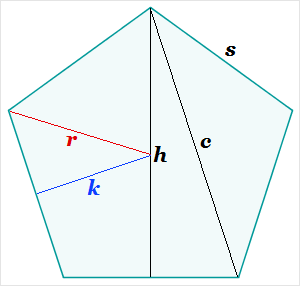# Pentagon Measurement Calculator

Pentagon Measurement Calculator
s =
h =
r =
k =
c =
area =
perimeter =
Select the radio button for one of the dimensions, enter its value, and click the calculate button to obtain the other measurementsThe side length, height, diagonal, center-to-side, center-to-vertex, and area of a regular pentagon are all interrelated. If you know one of these dimensions, you can compute the others.

For example, suppose the side length is s. If the height is h, the diagonal between non-adjacent vertices d, the distance from the side to the center k, the distance from the vertex to the center r, the area a, and the perimeter p, then you can find each measure with the following formulas:

r = s/[2*sin(π/5)]

h = r[1+cos(π/5)]

k = h - r

c = h/cos(π/10)

a = 2.5sk

p = 5s

Here, sin and cos are in radians, not degrees. (For conversion purposes, π/5 radians is 36°, and π/10 radians is 18°.)

You can perform these computations using a hand calculator, our online scientific calculator, or the convenient pentagonal measurement calculator at left. All results are accurate within 5 decimal places.